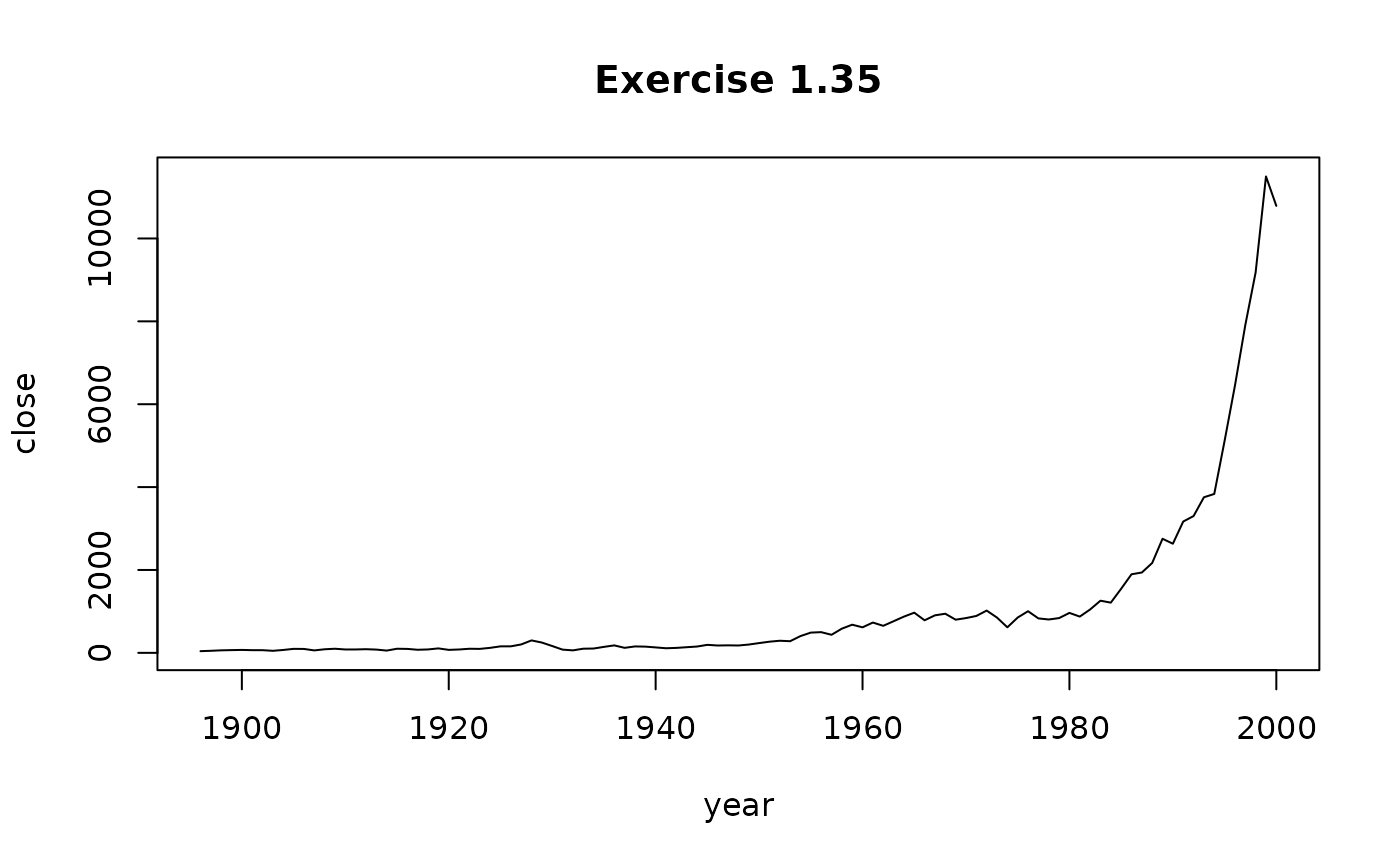Data for Exercise 1.35

Dowjones

## Format

A data frame/tibble with 105 observations on three variables

year

date

close

Dow Jones closing price

change

percent change from previous year

## Examples


plot(close ~ year, data = Dowjones, type = "l", main = "Exercise 1.35")if (FALSE) {
library(ggplot2)
ggplot2::ggplot(data = Dowjones, aes(x = year, y = close)) +
geom_point(size = 0.5) +
geom_line(color = "red") +
theme_bw() +
labs(y = "Dow Jones Closing Price")
}